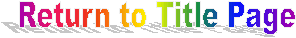Rebuttal to: Southwestern Oklahoma State University

New gravitational theory and equation explained.

Distinguished Professor, Ph.D. (name removed)
Science Education Section, Dept. of Chemistry and Physics
Southwestern Oklahoma State University
100 Campus Drive
Weatherford, OK 73096-3098

Ph.D.: I don't know why I received it, but out of curiosity I visited the URL address cited.  After only a cursory scan, I noticed a couple of errors in reasoning I felt I should bring to your attention (besides the numerous spelling errors, such as "breath" for "breadth" (corrected) and "it's" for "its".

Grant: The Grant Chronicles are meant to inform the scientific community of new ideas. Emphasis is placed on grammar and spelling, which has passed Microsoft’s Word spelling and grammar program.

Ph.D.: First, it is an error to substitute "gravitational force" for "pressure".  As you note, pressure is equal to force/area.  So force is used to calculate pressure, but cannot be substituted in its place.

Grant: You have overlooked the obvious; pressure is a force applied upon matter within a defined area. Gravity has the attributes of pressure as it applies a force upon all matter within the effected area. Isn’t gravity directly accountable for the containment pressure of the Sun according to present day theory? Thus stating, the fusion process of the Sun, which is responsible for the tremendous outward pressure of hot hydrogen –helium gases, is balanced by the force of gravity. How can one then turn around and say gravitation force and pressure on a mass cannot be substituted, when the Sun presently is a working example of this idea.

Ph.D.: Second, you seem to be confusing the universal gravitation constant (G) with the acceleration due to Earth's gravity (g).  The first, G, is part of the universal law of gravitation and is equal to approximately 6.67 x 10^-11 N-m^2/kg^2 (the units are Newton-meters squared per kilogram squared).  Clearly, this is NOT an acceleration and cannot be substituted for "a" in Newton's second law, F = ma.  The second, g, is the acceleration due to Earth's gravity and is equal to approximately 9.8 m/sec^2 (the units are meters per second squared or meters per second per second).  This quantity IS an acceleration and may be substituted into the equation F = ma to find the weight (W) of a mass (W = mg).

Grant: Addressing the confusion of the universal gravitation constant (G) with the acceleration due to Earth's gravity (g), I will set up the gravitational equation in comparison to force equation:

G * M1* M2  = Force                  Force  =  M *A

This Yeilds:

G * M1* M2      =          M *A

R2

M1 and M2 are factors of the total Mass so they cancel from both equations leaving:

G = A

R2

Conclusion: The gravitational constant G is the acceleration part in Newton’s F=M*A equation, but the constant R2 controls the magnitude of acceleration related to distance from the source. There is a direct relationship between the gravitational constant and acceleration with a function of G affected by R dictating the resultant A. Observations on Earth have shown that objects accelerate at the same rate and it is not dependent upon its mass. So if the mass can vary, force and acceleration move hand and hand. It is similar to putting a constant that proportionally affects another variable. Look at the affects of gravity, no matter the size of the mass, whether a feather or a canon ball in the absence of outside friction; they both fall at same the rate of acceleration.

Ph.D.: For the past few hundreds of years, thousands of brilliant scientists and mathematicians have studied the ideas you address on your web site.  Many have tried in vain to find flaws, because, after all, that is the way science advances.  None have been successful.  Good luck on your attempts.  I should warn you, however, that in my opinion attempts to support religious beliefs with scientific evidence are futile.  Beliefs are not based upon evidence or theories, but are based upon faith.  And scientific theories are not based upon beliefs, but upon evidence.  To mix the two, or to try to support one with the other, is a disservice to value of both science and religion.

Grant: Many scientists have found flaws. This is why science advances. The Grant Chronicles are not written to criticize or state that the current format of physics is wrong, but as mankind now embarks on a new path towards the future, the current ideas and theories are just the foundation. The Grant Chronicles is meant to bridge the gaps and introduce science to the next level.